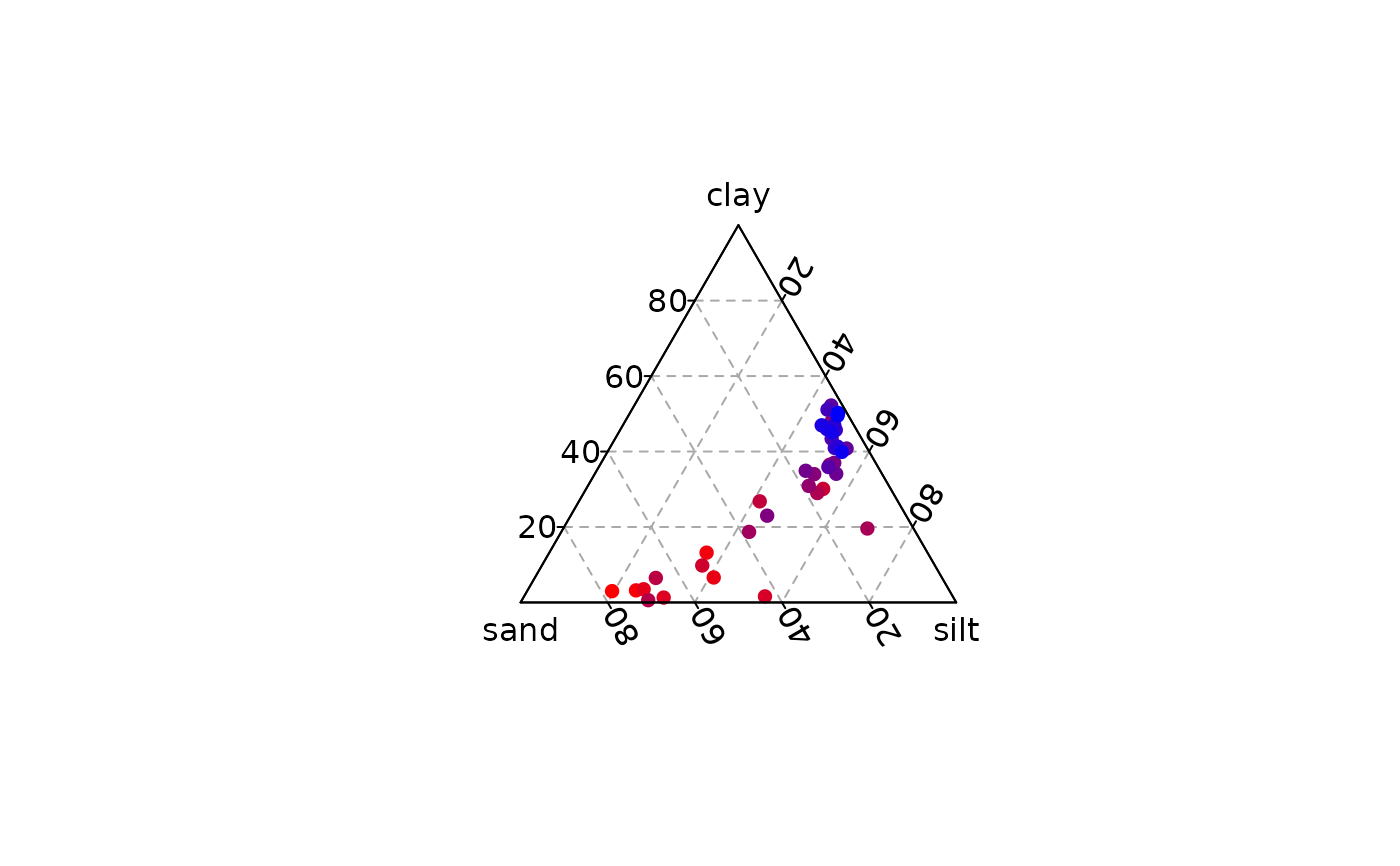Produces a ternary plot.

## Usage

ternary_plot(x, y, z, ...)

# S4 method for numeric,numeric,numeric
ternary_plot(
x,
y,
z,
xlim = NULL,
ylim = NULL,
zlim = NULL,
xlab = NULL,
ylab = NULL,
zlab = NULL,
main = NULL,
sub = NULL,
ann = graphics::par("ann"),
axes = TRUE,
frame.plot = axes,
panel.first = NULL,
panel.last = NULL,
...
)

# S4 method for ANY,missing,missing
ternary_plot(
x,
xlim = NULL,
ylim = NULL,
zlim = NULL,
xlab = NULL,
ylab = NULL,
zlab = NULL,
main = NULL,
sub = NULL,
ann = graphics::par("ann"),
axes = TRUE,
frame.plot = axes,
panel.first = NULL,
panel.last = NULL,
...
)

## Arguments

x, y, z

A numeric vector giving the x, y and z ternary coordinates of a set of points. If y and z are missing, an attempt is made to interpret x in a suitable way (see grDevices::xyz.coords()).

...

Other graphical parameters may also be passed as arguments to this function.

xlim

A length-two numeric vector giving the x limits in the range $$[0,1]$$.

ylim

A length-two numeric vector giving the y limits in the range $$[0,1]$$.

zlim

A length-two numeric vector giving the z limits in the range $$[0,1]$$.

xlab, ylab, zlab

A character string giving a label for the x, y and z axes.

main

A character string giving a main title for the plot.

sub

A character string giving a subtitle for the plot.

ann

A logical scalar: should the default annotation (title and x, y and z axis labels) appear on the plot?

axes

A logical scalar: should axes be drawn on the plot?

frame.plot

A logical scalar: should a box be drawn around the plot?

panel.first

An an expression to be evaluated after the plot axes are set up but before any plotting takes place. This can be useful for drawing background grids.

panel.last

An expression to be evaluated after plotting has taken place but before the axes, title and box are added.

## Value

ternary_plot() is called it for its side-effects: it results in a graphic being displayed.

Other graphical elements: ternary_axis(), ternary_box(), ternary_grid(), ternary_pairs(), ternary_title()

N. Frerebeau

## Examples

## Blank plot
ternary_plot(NULL)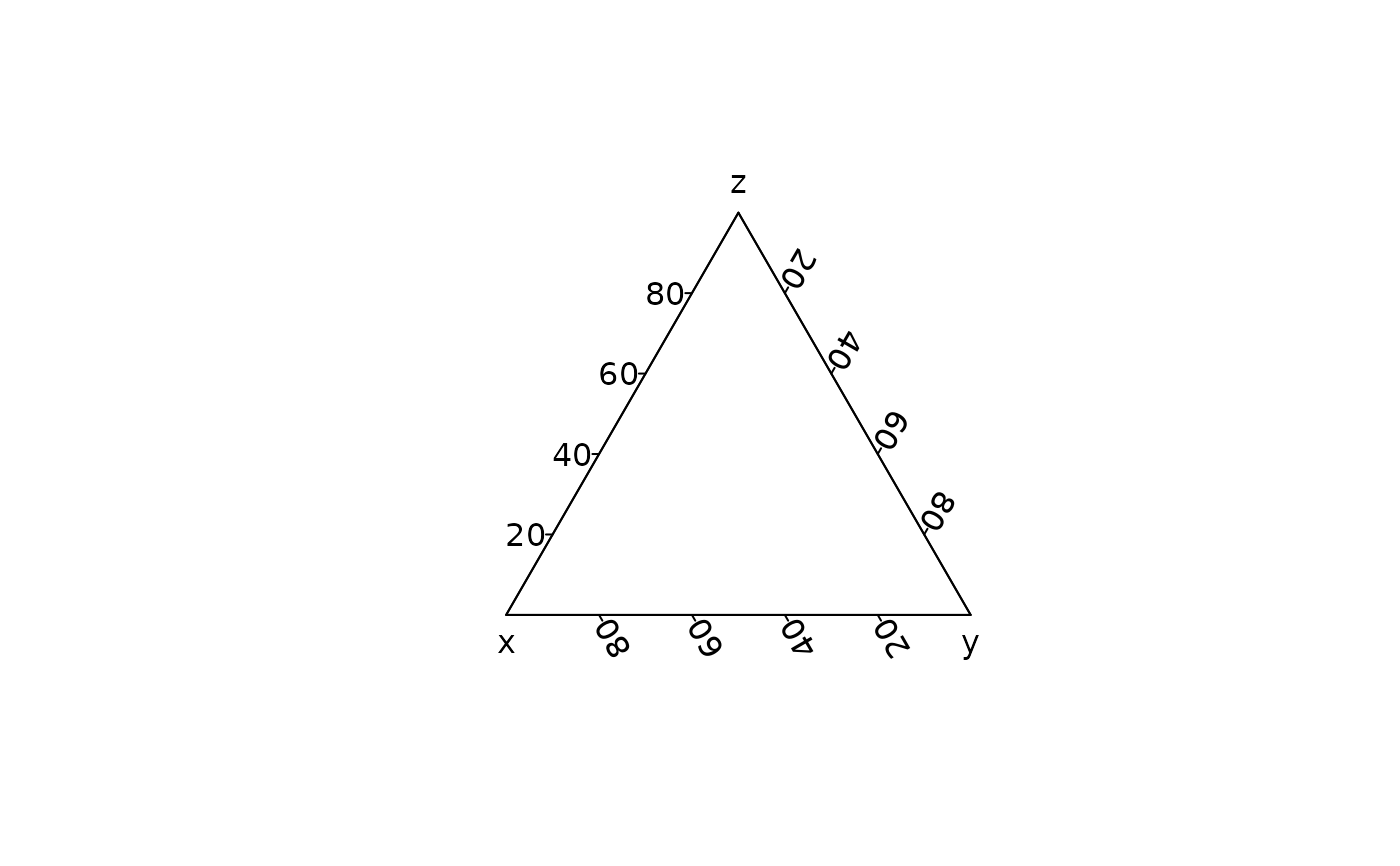## Compositional data
coda <- data.frame(
X = c(20, 60, 20, 1/3),
Y = c(20, 20, 60, 1/3),
Z = c(60, 20, 20, 1/3)
)

## Ternary plot
ternary_plot(coda, pch = 16, col = "red")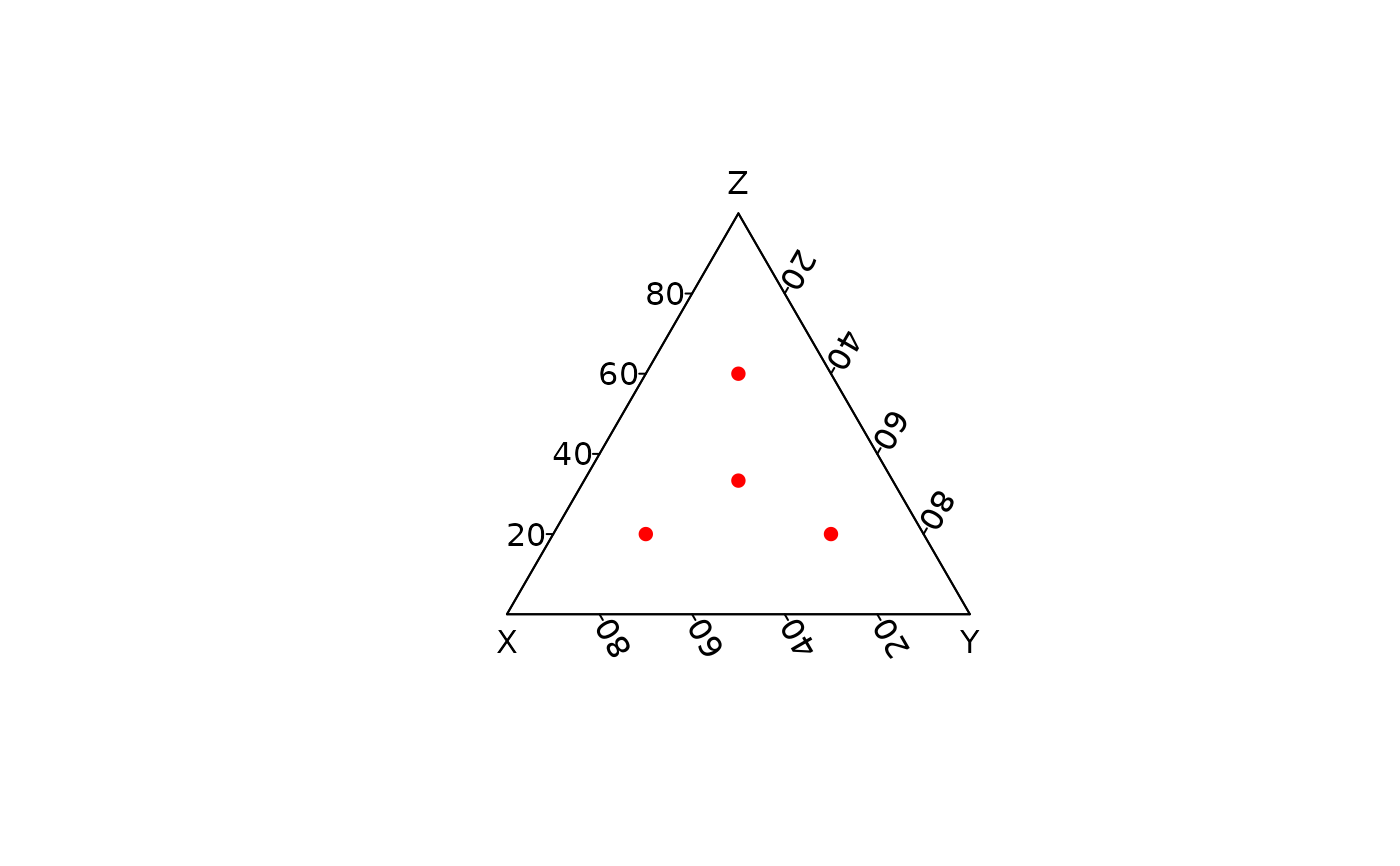ternary_plot(coda, panel.first = ternary_grid(5, 10))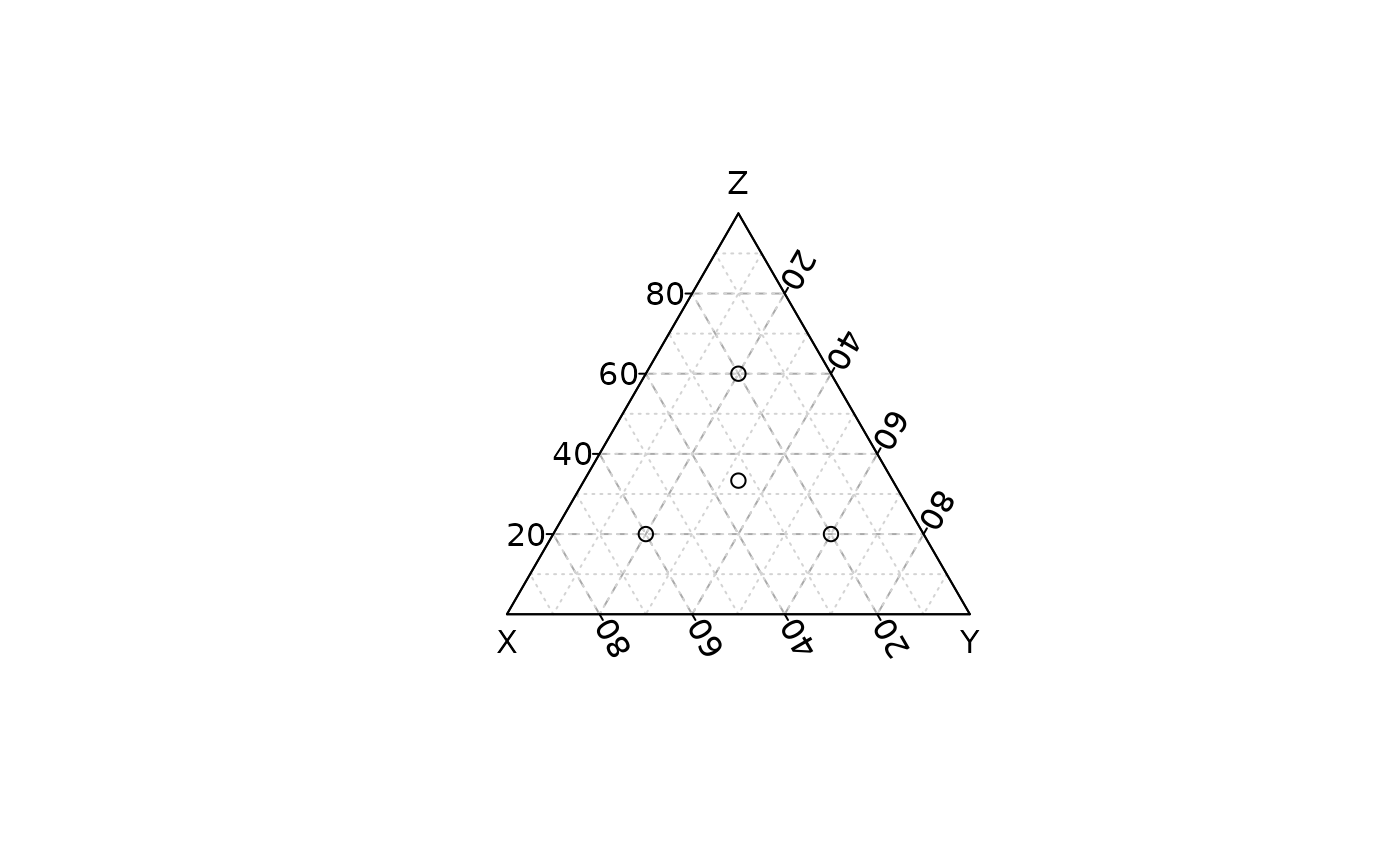## Zoom
ternary_plot(coda, xlim = c(0.5, 1), panel.first = ternary_grid())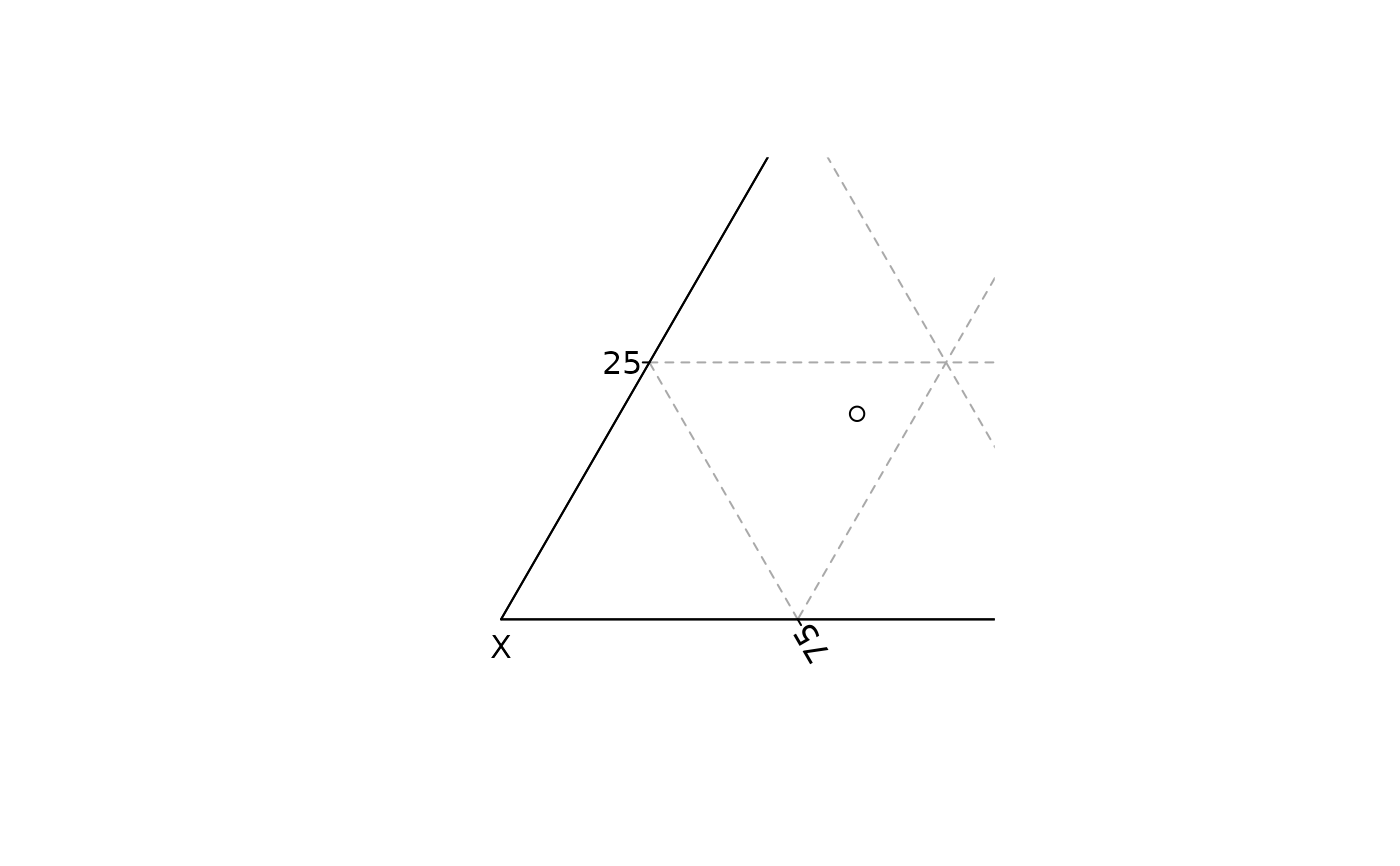ternary_plot(coda, ylim = c(0.5, 1), panel.first = ternary_grid())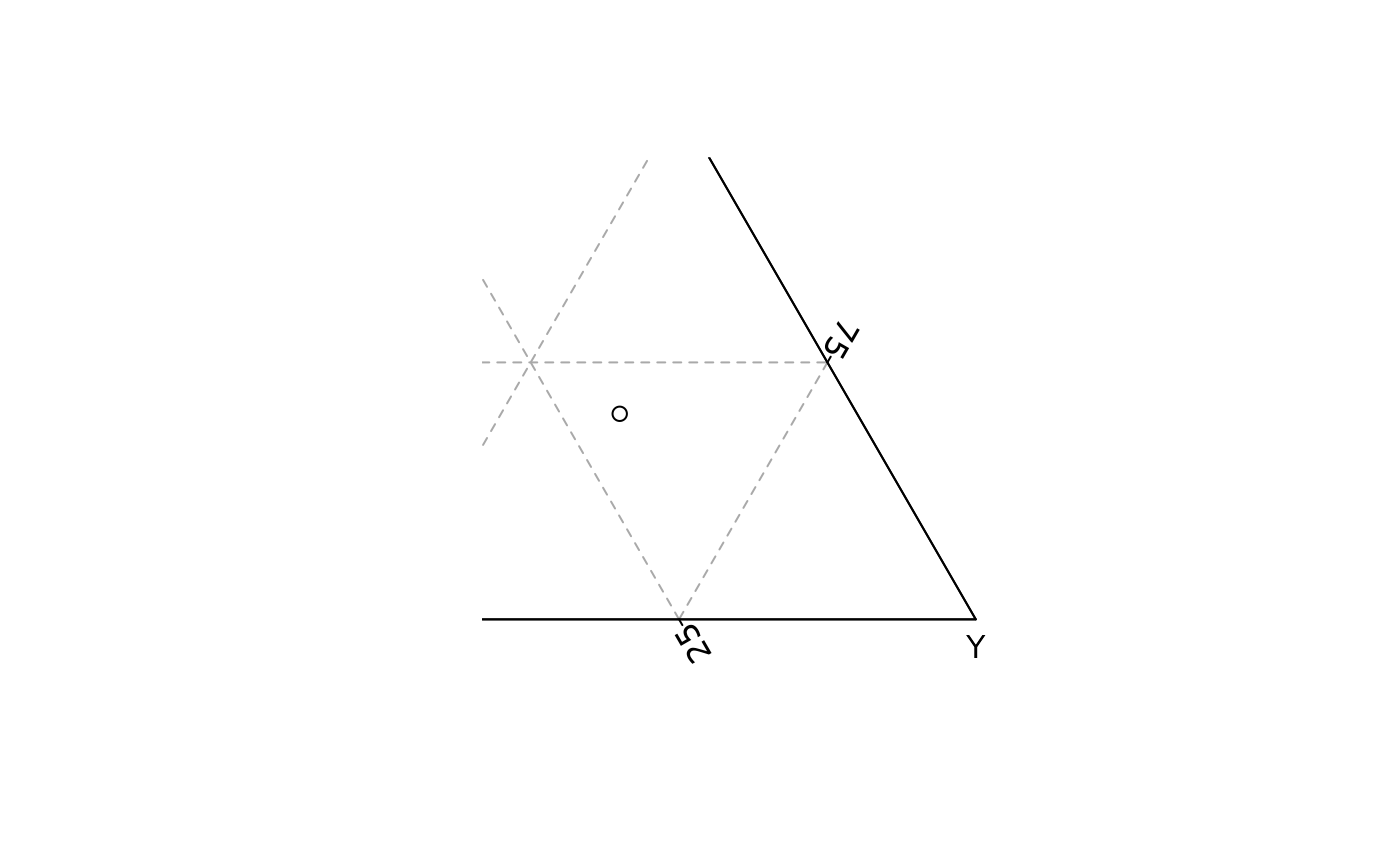ternary_plot(coda, zlim = c(0.5, 1), panel.first = ternary_grid())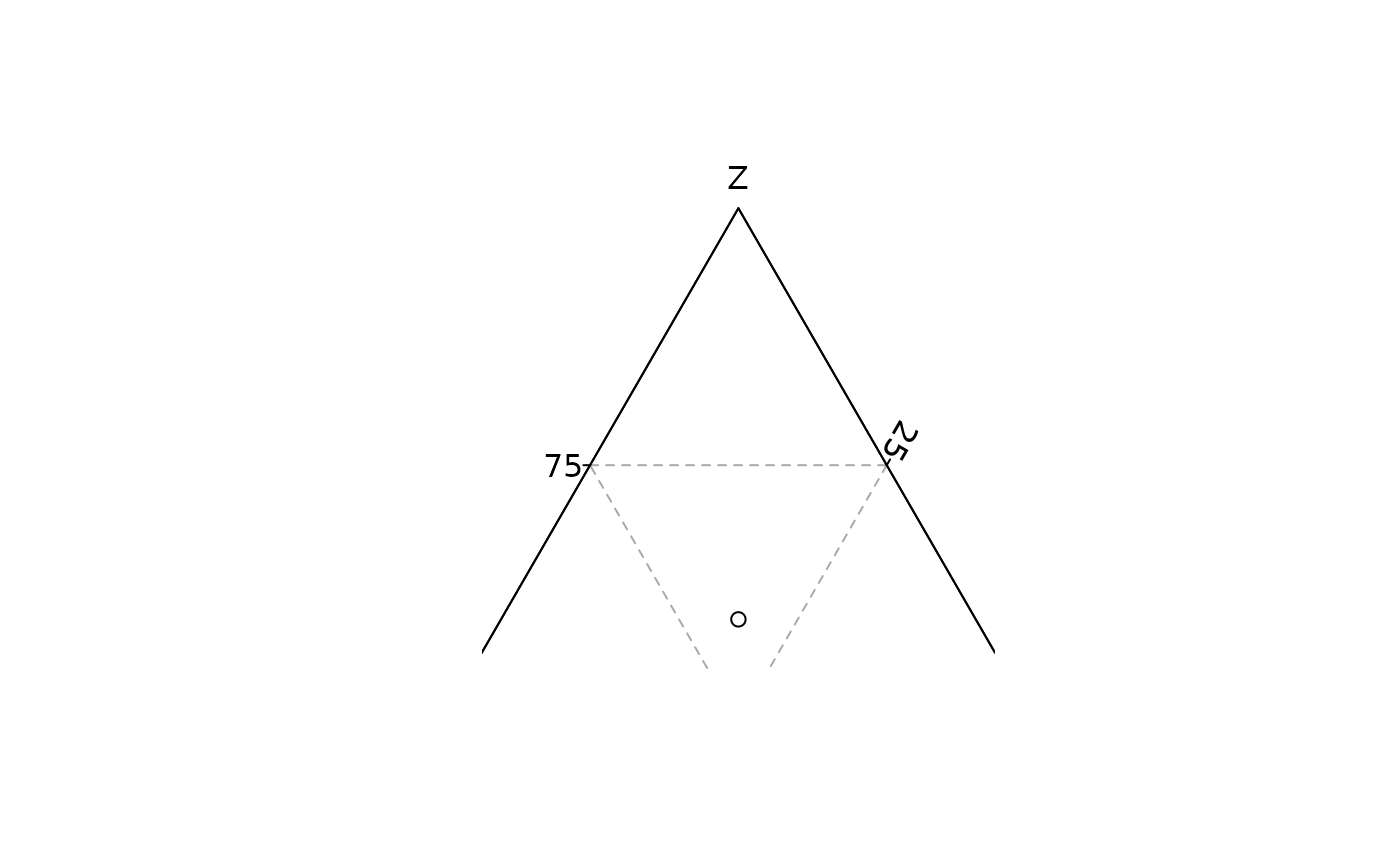## Color according to a supplementary variable
## Data from Aitchison 1986
col <- grDevices::colorRampPalette(c("red", "blue"))(nrow(arctic))
ternary_plot(arctic, panel.first = ternary_grid(), pch = 16, col = col)